# Java 1. (5) Assume selection sort is applied to the following array. Show the state of...

Java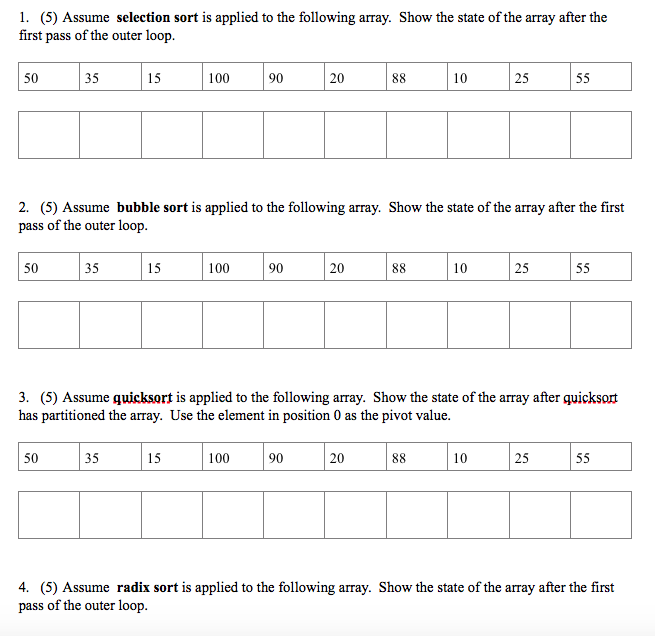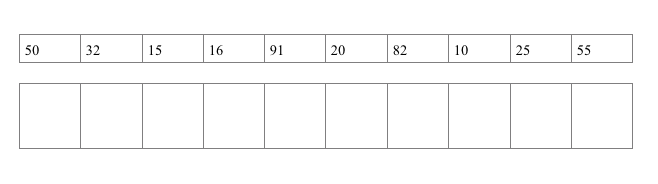1. (5) Assume selection sort is applied to the following array. Show the state of the array after the first pass of the outer loop. 50 35 15 100 90 20 10 25 2. (5) Assume bubble sort is applied to the following array. Show the state of the array after the first pass of the outer loop 50 35 15 100 90 20 10 25 3. (5) Assume quicksort is applied to the following array. Show the state of the array after quicksort has partitioned the array. Use the element in position 0 as the pivot value. 50 35 15 100 90 20 10 25 4. (5) Assume radix sort is applied to the following array. Show the state of the array after the first pass of the outer loop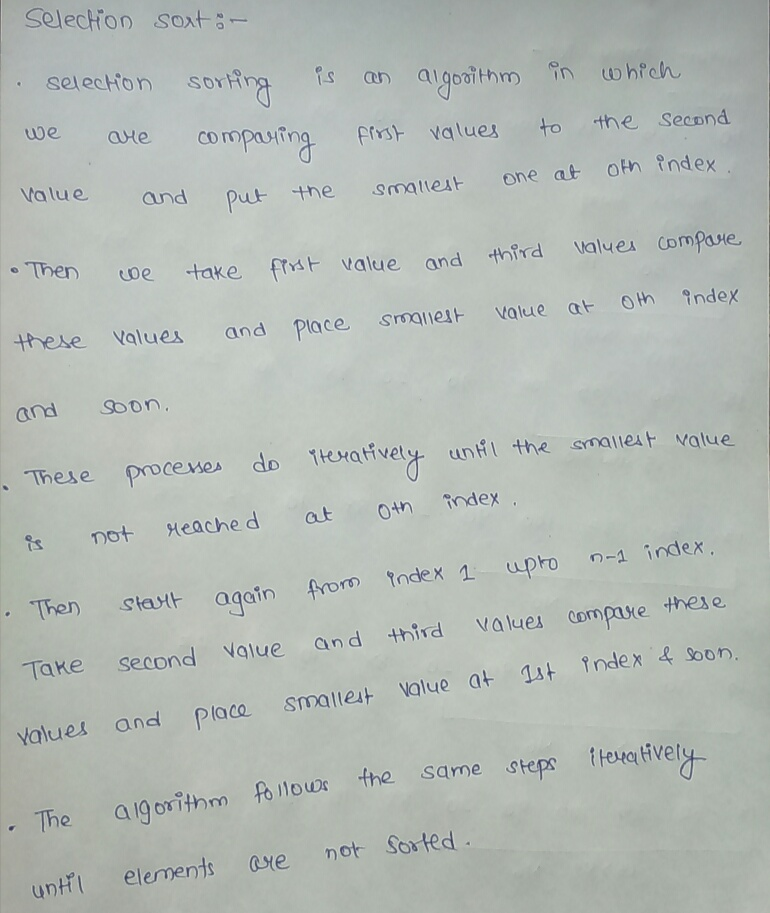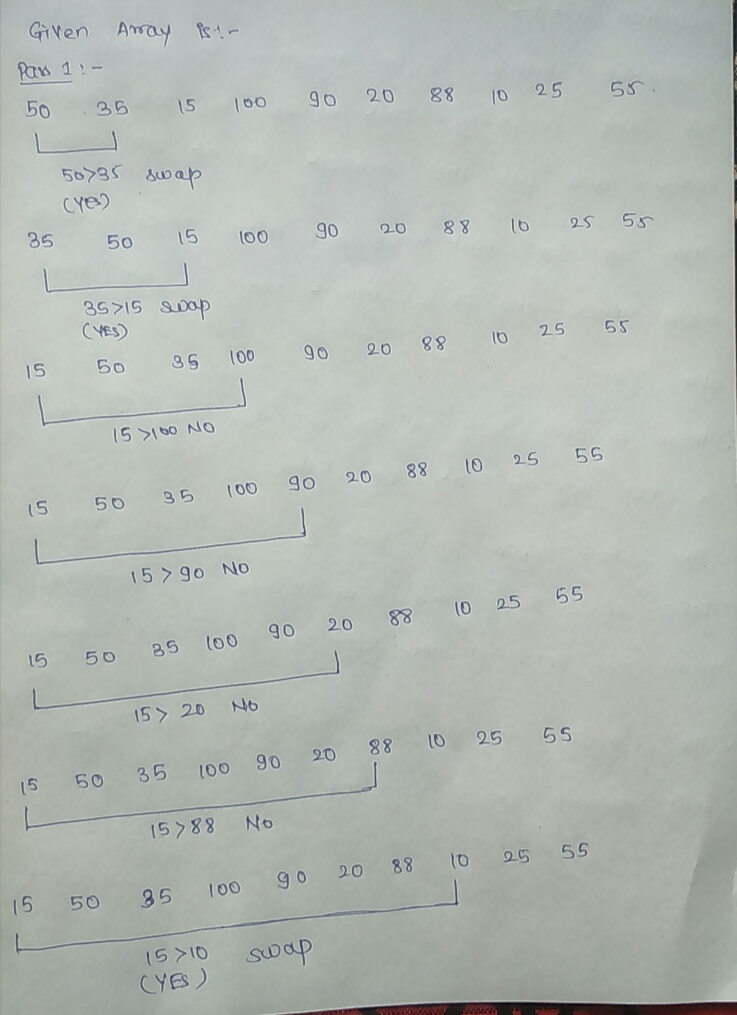up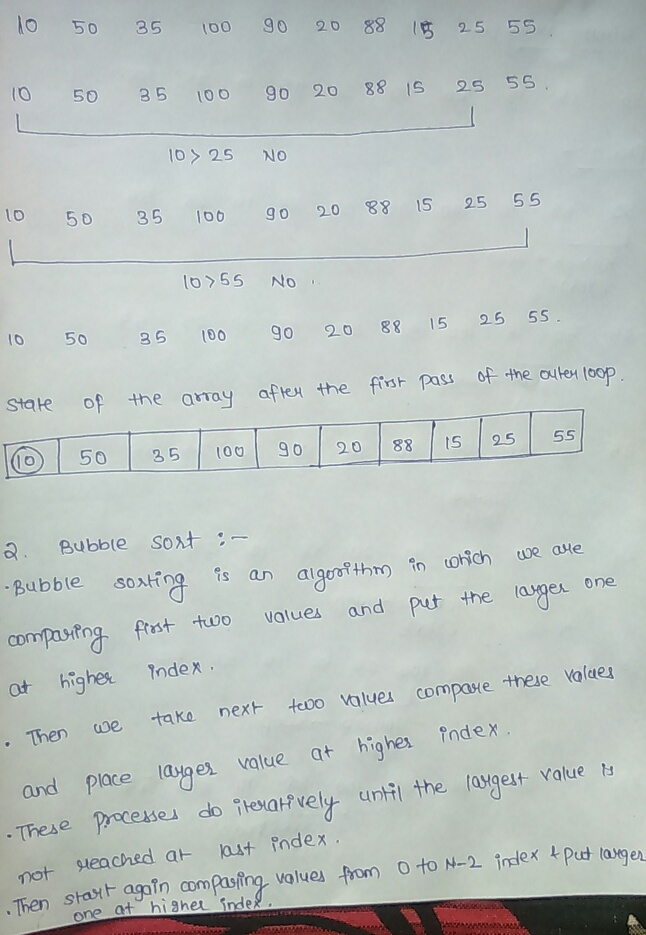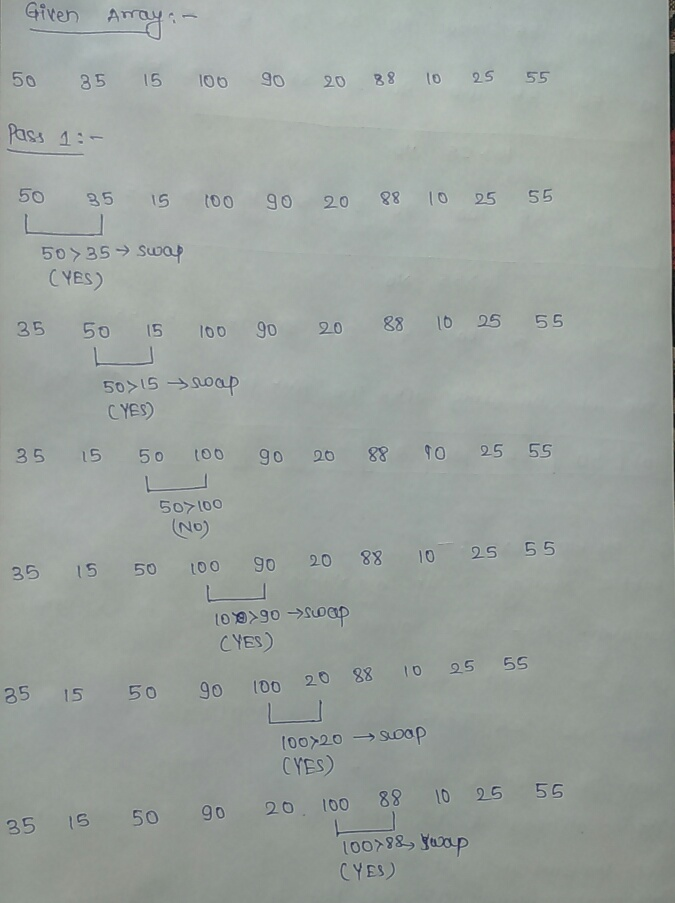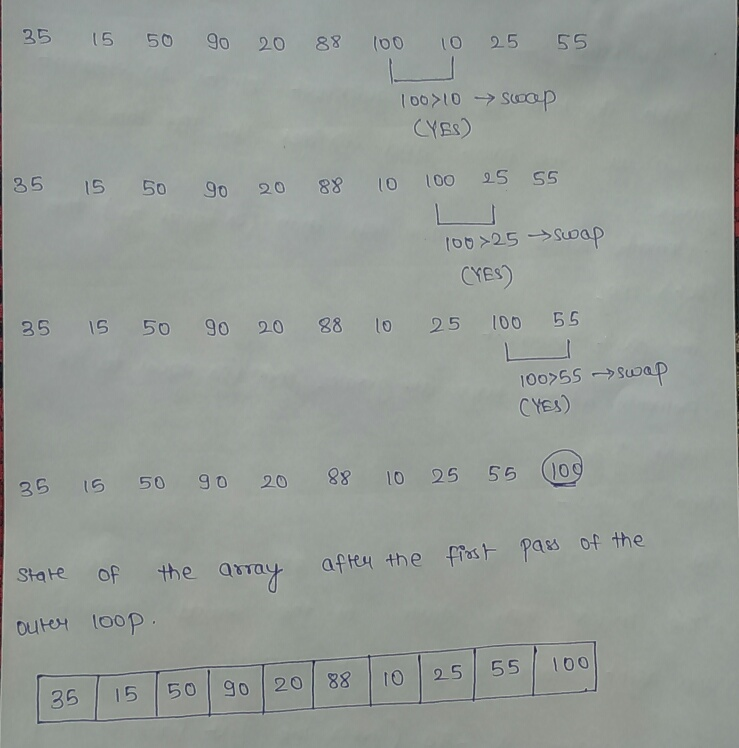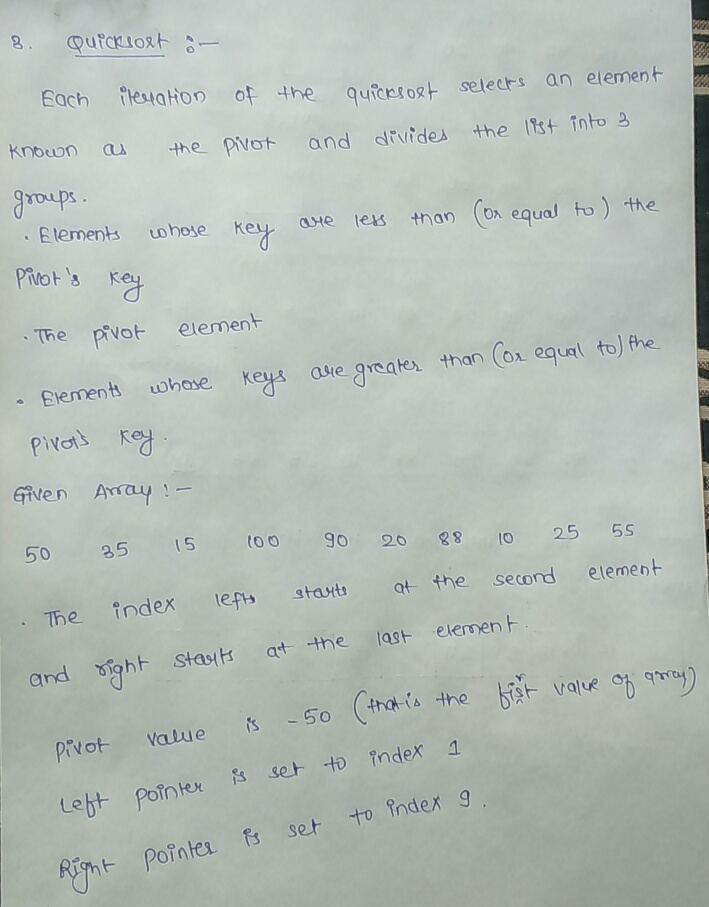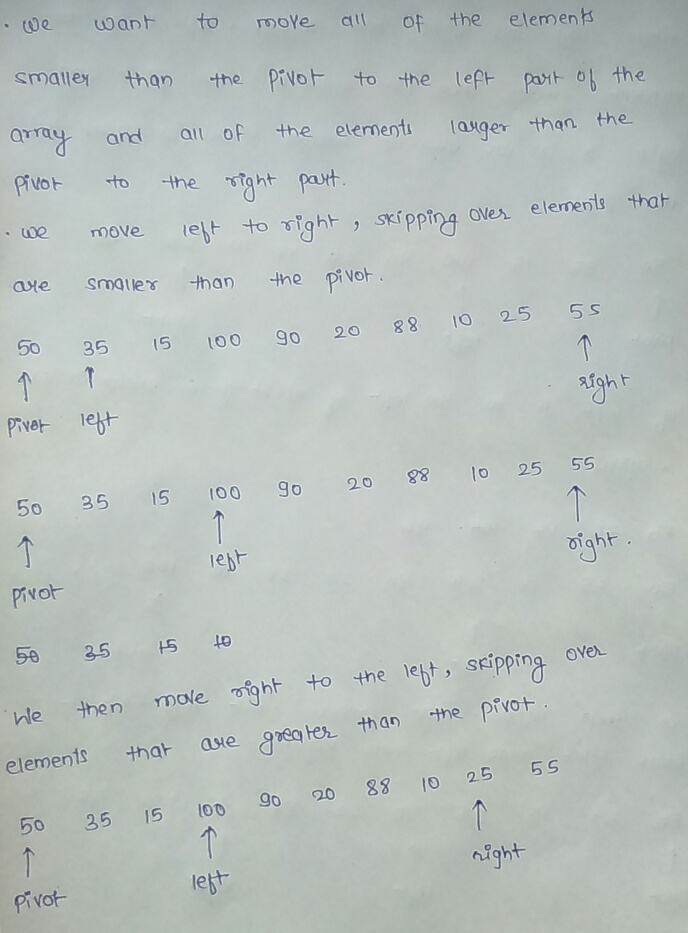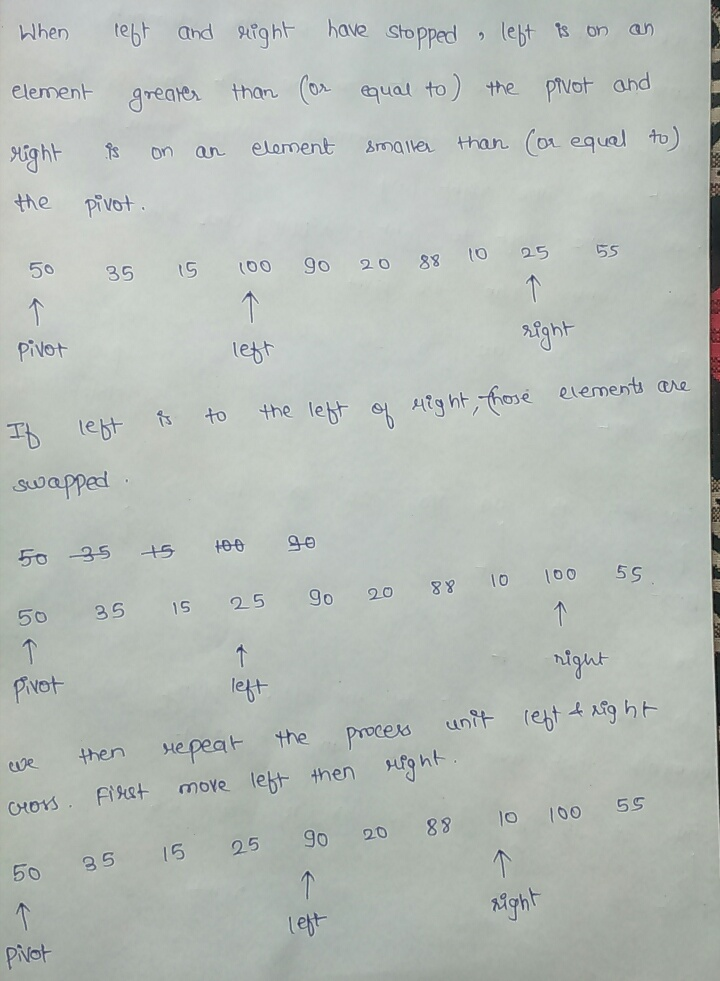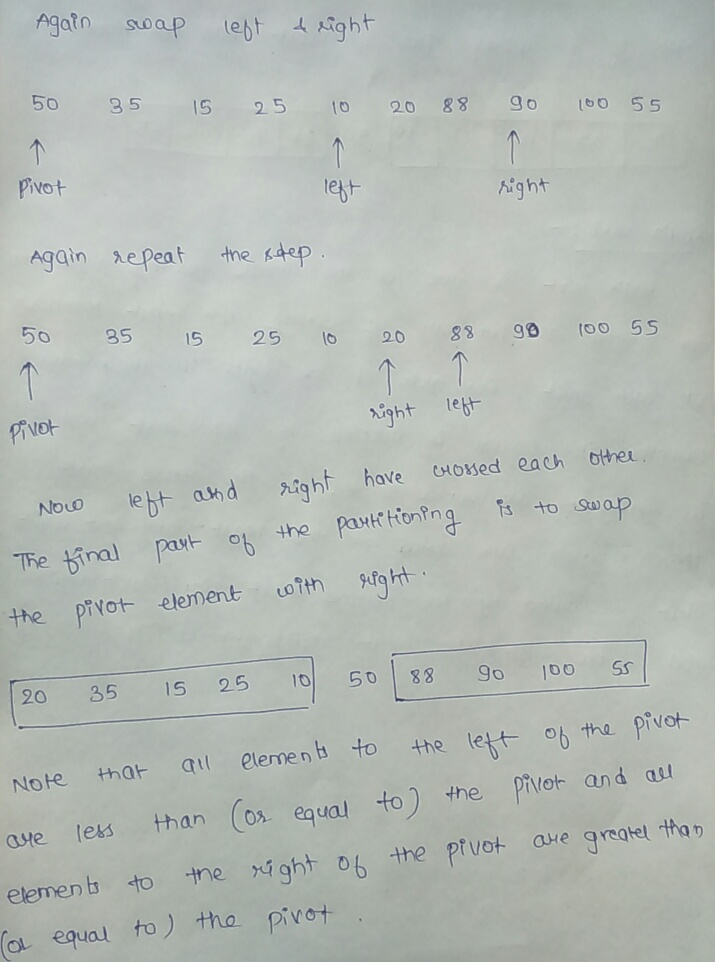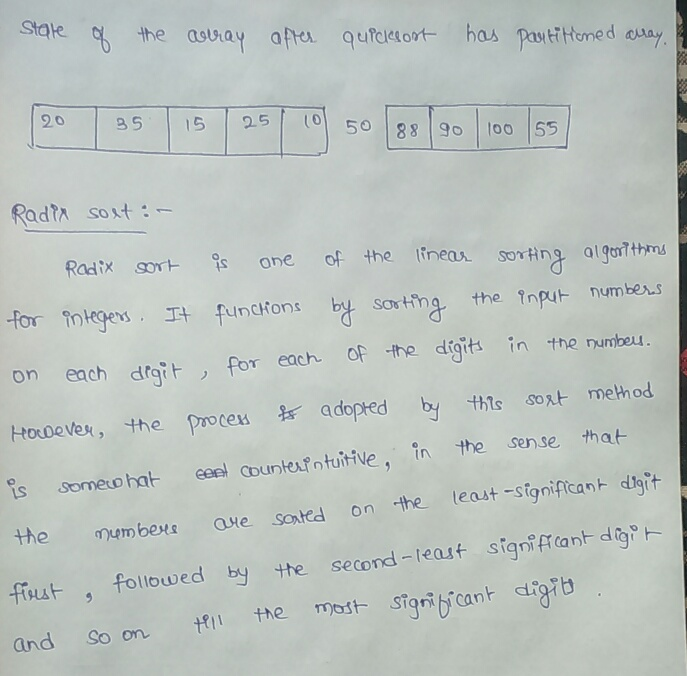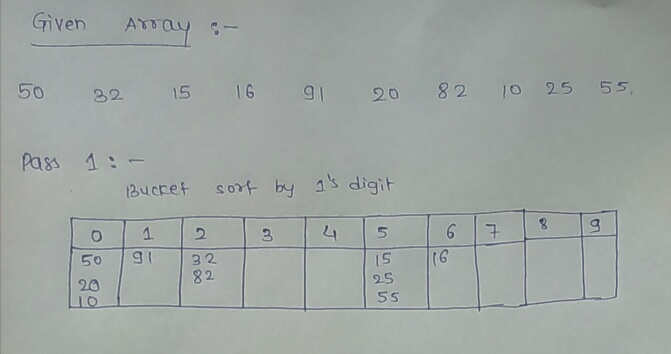#### Earn Coin

Coins can be redeemed for fabulous gifts.

Similar Homework Help Questions
• ### 5. Radix Sort Sort the following array using a radix sort with base 10, showing each...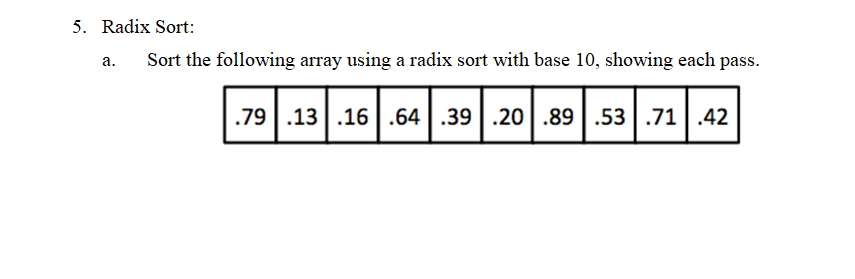5. Radix Sort Sort the following array using a radix sort with base 10, showing each pass a. .791316.64|.39|.20|.89.53|.71.42 5. Radix Sort Sort the following array using a radix sort with base 10, showing each pass a. .791316.64|.39|.20|.89.53|.71.42

• ### Sorting Sort the following array using the quick sort algorithm: (4 Marks) a. 12 26 8...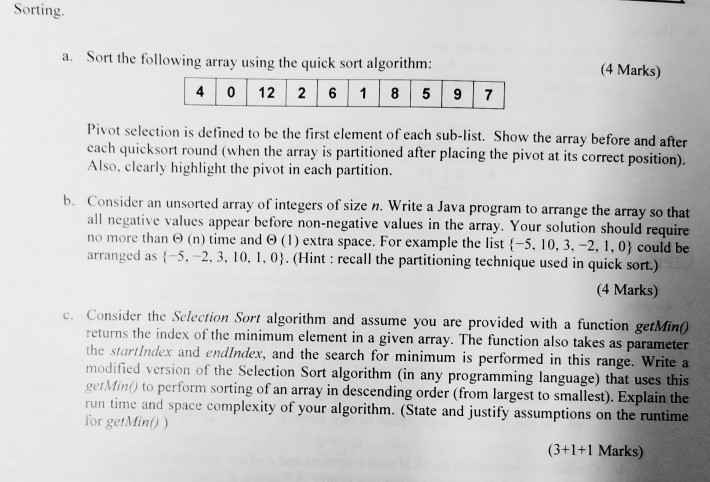Sorting Sort the following array using the quick sort algorithm: (4 Marks) a. 12 26 8 9 7 0 4 Pivot selection is defined to be the first element of each sub-list. Show the array before and after each quicksort round (when the array is partitioned after placing the pivot at its correct position). Also, clearly highlight the pivot in each partition b. Consider an unsorted array of integers of size n. Write a Java program to arrange the array...

• ### 5. Radix Sort Sort the following array using a radix sort with base 10, showing each...5. Radix Sort Sort the following array using a radix sort with base 10, showing each pass a. .791316.64|.39|.20|.89.53|.71.42

• ### JAVA Each of the following 6 questions refer to the following list of values in the...

JAVA Each of the following 6 questions refer to the following list of values in the order as shown. 555 288 633 666 444 422 399 477 21. Print the values in the order they would have after the first round of bubble sort’s bubbling operation low to high. 22. Print the values in the order they would have after the first round of selection sort for sorting low to high, assuming it selects the max. 23. Print the values...

• ### You want to sort (in increasing order) the following array of integers using quicksort as we...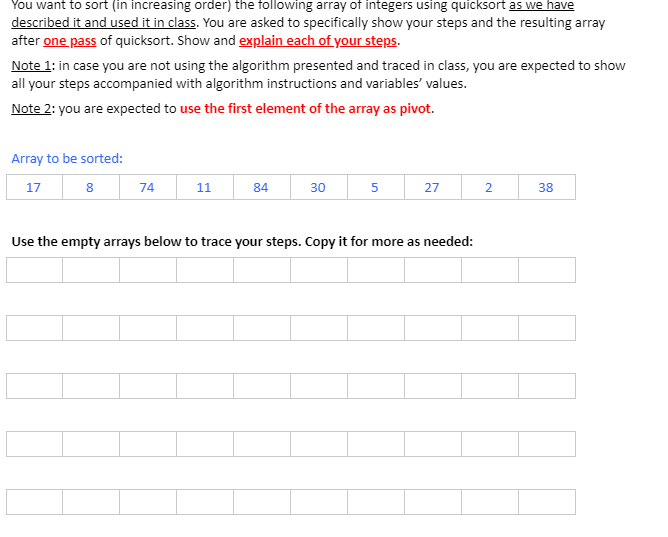You want to sort (in increasing order) the following array of integers using quicksort as we have described it and used it in class. You are asked to specifically show your steps and the resulting array after one pass of quicksort. Show and explain each of your steps. Note 1: in case you are not using the algorithm presented and traced in class, you are expected to show all your steps accompanied with algorithm instructions and variables' values. Note 2:...

• ### Sort the following list using the bubble sort algorithm as discussed in this chapter. Show the...

Sort the following list using the bubble sort algorithm as discussed in this chapter. Show the list after each iteration of the outer for loop. 46, 58, 16, 25, 83, 98, 8, 70, 5, 62

• ### Perform the partition method of quick sort once on the array [8, 12, 2, 15, 7]....

Perform the partition method of quick sort once on the array [8, 12, 2, 15, 7]. Show the array after each iteration of the while loop in the partition method. Use the first element (here it is 8) as the pivot. Show the two-sub array after one call to quick sort.

• ### PROBLEM #11 What are the two algorithms used to sort an array? How do they work?...

PROBLEM #11 What are the two algorithms used to sort an array? How do they work? -------------------------------------------------------------------- */ /* --------------------------------------------------------------------------------------------------------------- PROBLEM #12 Given the following array 'jumbled', what will the array be after the first swap of a selection sort algorithm? (Assume the algorithm sorts in ascending order). --------------------------------------------------------------------------------------------------------------- */ int jumbled[] = {12, 5, 3, 1, 9, 100, 45}; /* --------------------------------------------------------------------------------------------------------------- PROBLEM #13 Given the following array 'jumbled2', what will the array be after the third swap of a...

• ### Use Bubble Sort to Sort the following array. Show the steps you would take. [3, 1,...

Use Bubble Sort to Sort the following array. Show the steps you would take. [3, 1, 4, 1, 5, 9, 2, 6, 5]

• ### Sort the following array of integer using selection and insertion sort algorithms.             Notdoing the program . Show...

Sort the following array of integer using selection and insertion sort algorithms.             Notdoing the program . Show it step by step.             { 20   12   8   4   13   9   26   18   25   14}.

Free Homework App